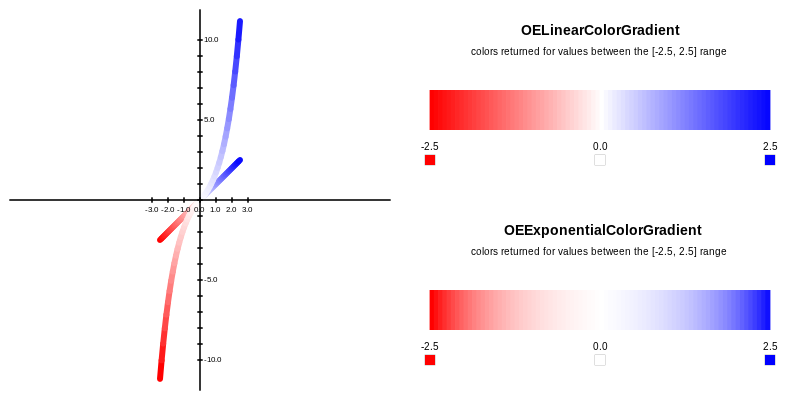class OEExponentialColorGradient : public OEColorGradientBase


The OEExponentialColorGradient class interpolates between colors that are defined by using the OEColorStop class. The OEExponentialColorGradient.TransformValue method defines the transformation that is performed before returning an interpolated color associated with a floating point value. The Figure: Example of linear and exponential color gradients illustrates the difference between a linear and a logarithmic colors gradients constructed with the same color stops.Example of linear and exponential color gradients generated with (-2.5, OERed), (0.0, OEWhite) and (+2.5, OEBlue) color stops

The following methods are publicly inherited from OEColorGradientBase:

## Constructors¶

OEExponentialColorGradient()


Default constructor that creates an “empty” color gradient.

Note

An “empty” color gradient always returns the OEBlack color, when calling the OEColorGradientBase.GetColorAt, the OEColorGradientBase.GetMinColor or the OEColorGradientBase.GetMaxColor methods.

OEExponentialColorGradient(const OEColorStop &oneStop)


Creates an OEExponentialColorGradient object with one color stop. An OEExponentialColorGradient object that has only one color stop will always return that color when calling the OEColorGradientBase.GetColorAt method.

OEExponentialColorGradient(const OEColorStop &bgnStop,
const OEColorStop &endStop)


Creates an OEExponentialColorGradient object with interpolation range between the two specified color stops.

OEExponentialColorGradient(const OEExponentialColorGradient &rhs)


Copy constructor.

## operator=¶

OEExponentialColorGradient &operator=(const OEExponentialColorGradient &rhs)


Assignment operator.

## TransformValue¶

double TransformValue(double value) const


Performs a $$f(x) = -exp(-x)+1.0$$ transformation when x is less than 0.0 and $$f(x) = exp(x)-1.0)$$ transformation when x is greater than 0.0. This method is called by OEColorGradientBase.GetColorAt